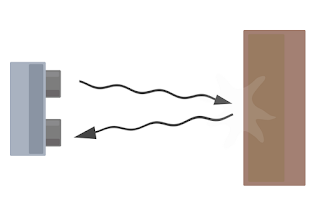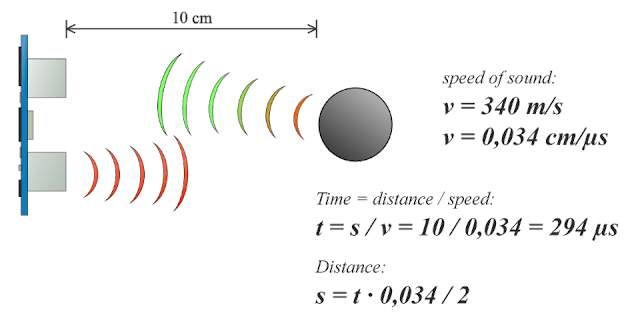Ultrasonic sensor( HC-SR04 with arduino) - LBM4

## Wednesday, 31 July 2019An Ultrasonic sensor is a device that can measure the distance to an object by using sound waves. It measures distance by sending out a sound wave at a specific frequency and listening for that sound wave to bounce back. By recording the elapsed time between the sound wave being generated and the sound wave bouncing back, it is possible to calculate the distance between the sonar sensor and the object.

### Distance Calculation:

Distance L = 1/2 × T × C

where L is the distance, T is the time between the emission and reception, and C is the sonic speed. (The value is multiplied by 1/2 because T is the time for go-and-return distance.)

## HC-SRO4

The HC-SR04 ultrasonic sensor uses sonar to determine distance to an object like bats do. It offers excellent non-contact range detection with high accuracy and stable readings in an easy-to-use package. It comes complete with ultrasonic transmitter and receiver modules.

### Features:

Here’s a list of some of the HC-SR04 ultrasonic sensor features and specials:

v Power Supply :+5V DC

v Quiescent Current : <2mA

v Working Current: 15mA
v Effectual Angle: <15°
v Ranging Distance : 2cm – 400 cm/1″ – 13ft
v Resolution : 0.3 cm
v Measuring Angle: 30 degree
v Trigger Input Pulse width: 10uS
v Dimension: 45mm x 20mm x 15mm

### Working Procedure:

It emits an ultrasound at 40 000 Hz which travels through the air and if there is an object or obstacle on its path It will bounce back to the module. Considering the travel time and the speed of the sound you can calculate the distance.

The HC-SR04 Ultrasonic Module has 4 pins, Ground, VCC, Trig and Echo. The Ground and the VCC pins of the module needs to be connected to the Ground and the 5 volts pins on the Arduino Board respectively and the trig and echo pins to any Digital I/O pin on the Arduino Board.In order to generate the ultrasound you need to set the Trig on a High State for 10 µs. That will send out an 8 cycle sonic burst which will travel at the speed sound and it will be received in the Echo pin. The Echo pin will output the time in microseconds the sound wave traveled.For example, if the object is 10 cm away from the sensor, and the speed of the sound is 340 m/s or 0.034 cm/µs the sound wave will need to travel about 294 u seconds. But what you will get from the Echo pin will be double that number because the sound wave needs to travel forward and bounce backward.  So in order to get the distance in cm we need to multiply the received travel time value from the echo pin by 0.034 and divide it by 2.## Arduino with HC-SRO4 Sensor:

This sensor is very popular among the Arduino tinkerers. So, here we provide an example on how to use the HC-SR04 ultrasonic sensor with the Arduino. In this project the ultrasonic sensor reads and writes the distance to an object in the serial monitor.

The goal of this project is to help you understand how this sensor works. Then, you should be able to use this example in your own projects.

Note: There’s an Arduino library called NewPing that can make your life easier when using this sensor.

The following table shows the connections you need to make:

 Ultrasonic Sensor HC-SR04 Arduino VCC 5V Trig Pin 11 Echo Pin 12 GND GND

## CODE

``````/*
* created by Rui Santos, https://randomnerdtutorials.com
*
* Complete Guide for Ultrasonic Sensor HC-SR04
*
Ultrasonic sensor Pins:
VCC: +5VDC
Trig : Trigger (INPUT) - Pin11
Echo: Echo (OUTPUT) - Pin 12
GND: GND
*/

int trigPin = 11;    // Trigger
int echoPin = 12;    // Echo
long duration, cm, inches;

void setup() {
//Serial Port begin
Serial.begin (9600);
//Define inputs and outputs
pinMode(trigPin, OUTPUT);
pinMode(echoPin, INPUT);
}

void loop() {
// The sensor is triggered by a HIGH pulse of 10 or more microseconds.
// Give a short LOW pulse beforehand to ensure a clean HIGH pulse:
digitalWrite(trigPin, LOW);
delayMicroseconds(5);
digitalWrite(trigPin, HIGH);
delayMicroseconds(10);
digitalWrite(trigPin, LOW);

// Read the signal from the sensor: a HIGH pulse whose
// duration is the time (in microseconds) from the sending
// of the ping to the reception of its echo off of an object.
pinMode(echoPin, INPUT);
duration = pulseIn(echoPin, HIGH);

// Convert the time into a distance
cm = (duration/2) / 29.1;     // Divide by 29.1 or multiply by 0.0343
inches = (duration/2) / 74;   // Divide by 74 or multiply by 0.0135

Serial.print(inches);
Serial.print("in, ");
Serial.print(cm);
Serial.print("cm");
Serial.println();

delay(250);
}``````

## Working Of Code:

First, variables for the trigger and echo pin called trigPin and echoPin should be created, respectively. The trigger pin is connected to digital Pin 11and the echo pins is connected to digital Pin 12:
``````int trigPin = 11;
int echoPin = 12;``````
You also create three variables of type long: durationcm and inch. The duration variable saves the time between the emission and reception of the signal. The cm variable will save the distance in centimeters, and the inch variable will save the distance in inches.
``long duration, cm, inches;``
In the setup(), initialize the serial port at a baud rate of 9600, and set the trigger pin as an output and the echo pin as an input.
``````//Serial Port begin
Serial.begin (9600);
//Define inputs and outputs
pinMode(trigPin, OUTPUT);
pinMode(echoPin, INPUT);``````
In the loop(), trigger the sensor by sending a HIGH pulse of 10 microseconds. But, before that, give a short LOW pulse to ensure you’ll get a clean HIGH pulse:
``````digitalWrite(trigPin, LOW);
delayMicroseconds(5);
digitalWrite(trigPin, HIGH);
delayMicroseconds(10);
digitalWrite(trigPin, LOW);``````
Then, you can read the signal from the sensor – a HIGH pulse whose duration is the time in microseconds from the sending of the signal to the reception of its echo to an object.
``duration = pulseIn(echoPin, HIGH)``
Finally, you just need to convert the duration to a distance. We can calculate the distance by using the following formula:
```distance = (traveltime/2) x speed of sound

The speed of sound is: 343m/s = 0.0343 cm/uS = 1/29.1 cm/uS

Or in inches: 13503.9in/s = 0.0135in/uS = 1/74in/uS```
We need to divide the traveltime by 2 because we have to take into account that the wave was sent, hit the object, and then returned back to the sensor.
``````cm = (duration/2) / 29.1;
inches = (duration/2) / 74;``````
Finally, we print the results in the Serial Monitor:
``````Serial.print(inches);
Serial.print("in, ");
Serial.print(cm);
Serial.print("cm");
Serial.println();``````